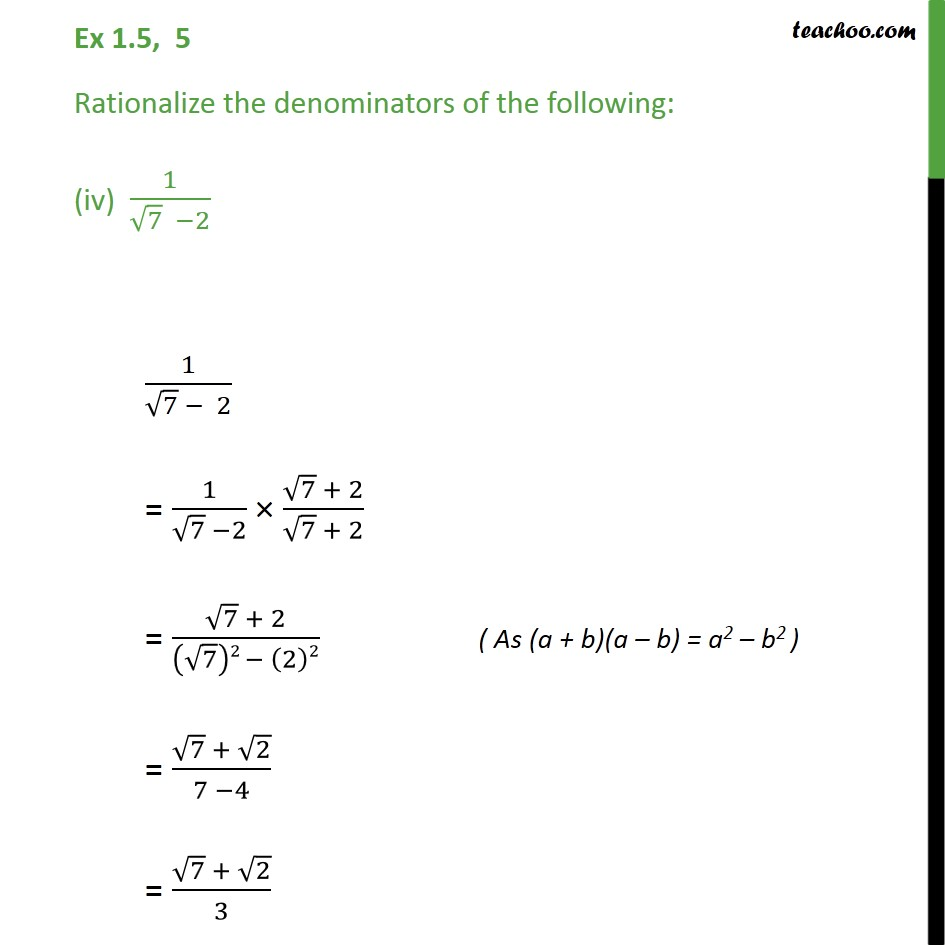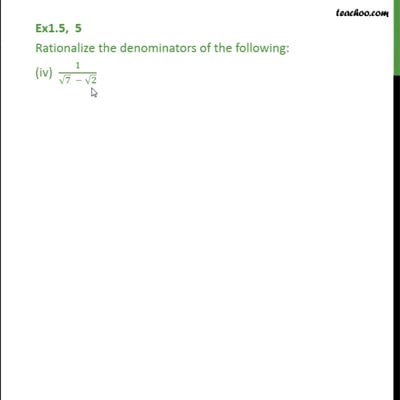Ex 1.5

Chapter 1 Class 9 Number Systems
Serial order wiseThis video is only available for Teachoo black users

Introducing your new favourite teacher - Teachoo Black, at only ₹83 per month

### Transcript

Ex 1.5, 5 Rationalize the denominators of the following: (iv) 1/(√7 −2) 1/(√7 − 2) = 1/(√7 −2) × (√7 + 2)/(√7 + 2) = (√7 + 2)/((√7)2 − (2)2) ( As (a + b)(a – b) = a2 – b2 ) = (√7 + √2)/(7 −4) = (√7 + √2)/3